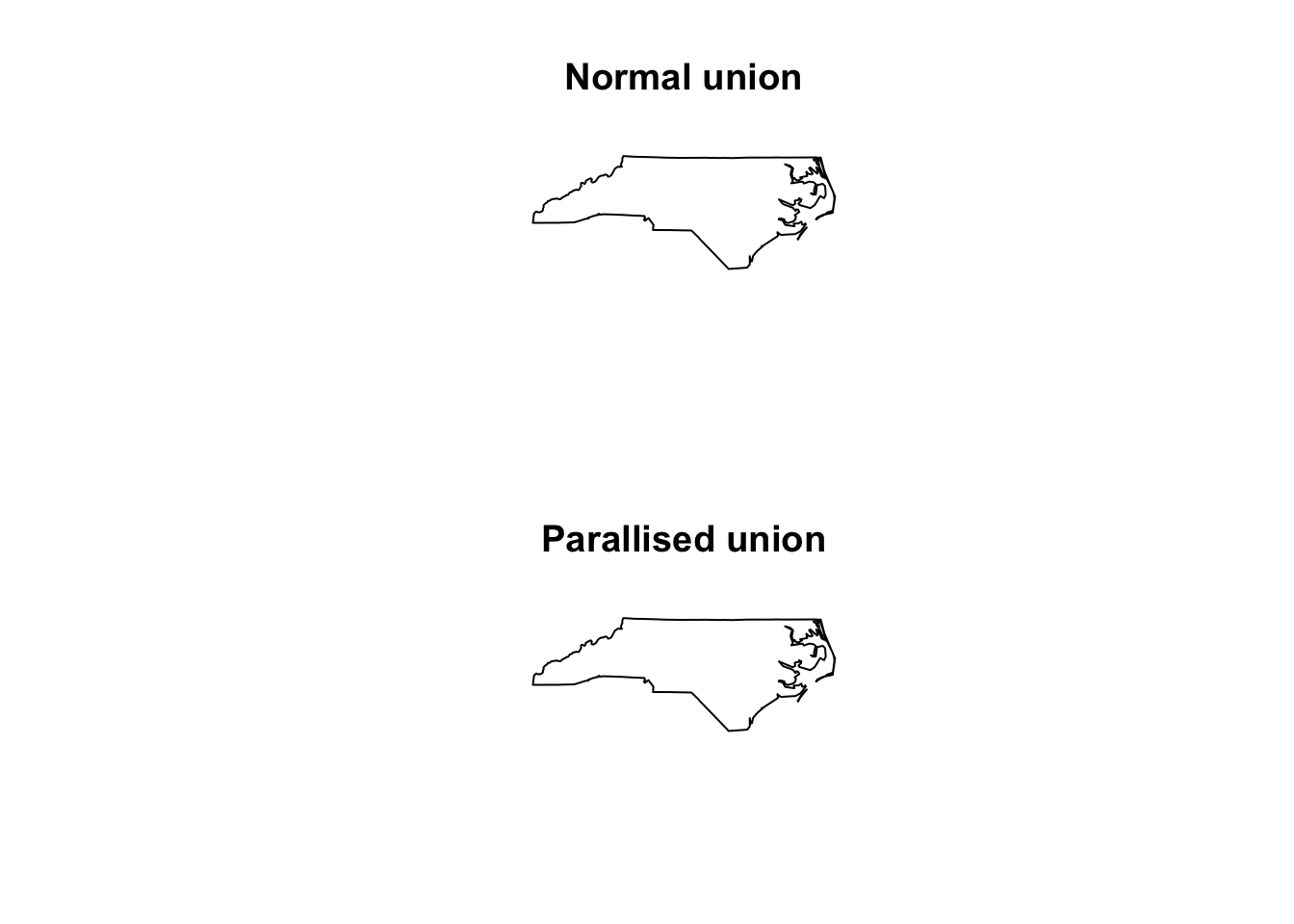# Spatial Analytics

Geography, some economics and data analysis.

# Fixing st_par to st_parallel

### 2018-04-01

Well, on September 27th, 2018, my first daughter arrived. Subsequently, my grand plans for blogging quickly diminished as my life became consumed by nappies and other babyish things. She had a rough start but is settling in now. In the meantime, Nathan Paul Mietkiewicz has been trying to use my st_par() function that I wrote about in this post but unfortunately it breaks when passed a st_union call. Let’s have a look at fixing the issues.

The original st_par function looks like:

# Paralise any simple features analysis.
st_par <- function(sf_df, sf_func, n_cores, ...){

# Create a vector to split the data set up by.
split_vector <- rep(1:n_cores, each = nrow(sf_df) / n_cores, length.out = nrow(sf_df))

# Perform GIS analysis
split_results <- split(sf_df, split_vector) %>%
mclapply(function(x) sf_func(x, ...), mc.cores = n_cores)

# Combine results back together. Method of combining depends on the output from the function.
if ( class(split_results[]) == 'list' ){
result <- do.call("c", split_results)
names(result) <- NULL
} else {
result <- do.call("rbind", split_results)
}

# Return result
return(result)
}

The problem is that st_union returns a ‘sfc’ object or simple features column. This is still a list, but it is a different ‘class’. For example:

nc_union <- st_union(nc)
class(nc_union)
##  "sfc_MULTIPOLYGON" "sfc"
mode(nc_union)
##  "list"

So that when the first if failed, it went straight to ‘rbind’ expecting a dataframe. In all honesty, this was rather poor programming! However the fix is simple (note that I renamed the function st_parallel):

# Paralise any simple features analysis.
st_parallel <- function(sf_df, sf_func, n_cores, ...){

# Create a vector to split the data set up by.
split_vector <- rep(1:n_cores, each = nrow(sf_df) / n_cores, length.out = nrow(sf_df))

# Perform GIS analysis
split_results <- split(sf_df, split_vector) %>%
parallel::mclapply(function(x) sf_func(x, ...), mc.cores = n_cores)

# Define the output_class. If length is greater than two, then grab the second variable.
output_class <- class(split_results[])
if (length(output_class) == 2){
output_class <- output_class
}

# Combine results back together. Method of combining depends on the output from the function.
if (output_class == "matrix"){
result <- do.call("rbind", split_results)
names(result) <- NULL
} else if (output_class == "sfc") {
result <- do.call("c", split_results)
result <- sf_func(result) # do.call combines the list but there are still n_cores of the geometry which had been split up. Running st_union or st_collect gathers them up into one, as is the expected output of these two functions.
} else if (output_class %in% c('list', 'sgbp') ){
result <- do.call("c", split_results)
names(result) <- NULL
} else if (output_class == "data.frame" ){
result <- do.call("rbind", split_results)
} else {
stop("Unknown class. st_parallel only accepts the following outputs at present: sfc, list, sf, matrix, sgbp.")
}

# Return result
return(result)
}

I’ve also tidied up the behaviour of the function to emit an error when it receives a class it hasn’t been built to handle yet. Let’s run it:

nc_union_par <- st_parallel(nc, st_union, 3)

par(mfrow = c(2,1))
plot(nc_union, main = "Normal union")
plot(nc_union_par, main = "Parallised union")Let’s check the other functions to make sure they’re still working:

sf_df_1 <- matrix(rnorm(12),nc=2) %>% data.frame %>% st_as_sf(coords = c("X1", "X2"))
sf_df_2 <- matrix(rnorm(12),nc=2) %>% data.frame %>% st_as_sf(coords = c("X1", "X2"))
distance_par <- st_parallel(sf_df_1, st_distance, 3, y = sf_df_2)
distance_normal <- st_distance(sf_df_1, sf_df_2)
identical(distance_normal, distance_par)
##  TRUE

Great! Now for an intersects:

# Buffer points
sf_df_1_buff <- st_buffer(sf_df_1, 1)
sf_df_2_buff <- st_buffer(sf_df_2, 1)

# Run parallel intersection
intersects_par <- st_parallel(sf_df_1_buff, st_intersects, 3, y = sf_df_2_buff)
intersects_par
## []
##  1 2 3 4 6
##
## []
##  1 3 5 6
##
## []
##  4
##
## []
##  1 2 3 4 6
##
## []
##  1 2 3 4 6
##
## []
##  1 3 5 6

Unfortunately, the st_intersects returns a new class called ‘sgbp’ which is dropped once it is passed through the call to ‘do.call’. However, the result is still essentially in that it is a list.

intersection_par <- st_parallel(sf_df_1_buff, st_intersection, 3, y = sf_df_2_buff)
intersection_par
## Simple feature collection with 24 features and 0 fields
## geometry type:  POLYGON
## dimension:      XY
## bbox:           xmin: -1.068456 ymin: -2.32419 xmax: 1.994464 ymax: 2.091797
## epsg (SRID):    NA
## proj4string:    NA
## First 10 features:
##                          geometry
## 1  POLYGON ((0.2542789 -0.2203...
## 2  POLYGON ((-0.2769673 -1.888...
## 3  POLYGON ((1.312677 0.777802...
## 4  POLYGON ((0.5494241 -0.1934...
## 5  POLYGON ((-0.2373857 -2.006...
## 6  POLYGON ((1.312677 0.777802...
## 7  POLYGON ((-0.03220291 -2.32...
## 8  POLYGON ((1.312677 0.777802...
## 9  POLYGON ((0.2767354 -0.7221...
## 10 POLYGON ((0.5209957 -0.7881...

Yay. I should really go through all of the outputs from sf functions, but I must go change a nappy…